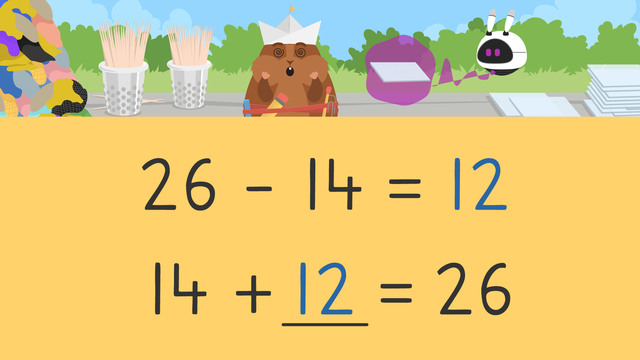# Subtract Using Addition on a Number Line

Content Subtract Using Addition on a Number LineRating

Ø 5.0 / 4 ratings
The authorsTeam Digital
Subtract Using Addition on a Number Line
CCSS.MATH.CONTENT.2.OA.A.1

## Basics on the topicSubtract Using Addition on a Number Line

When solving subtraction problems, you can us addition to subtract. There are many tools and strategies to solve addition problems, one of which is using a number line! When using addition to solve subtraction problems...

• Start by creating a new addition equation using what you know from the subtraction equation.

• Next, put the smaller addend on the left side of the number line.

• Then, count up the bigger number in the equation. You can do this by counting forward small amounts, like by fives or tens!

• Finally, find your sum by looking at how much you counted to get to the second number. Remember, the answer to the addition equation is also the answer to your subtraction equation!

## Subtract Using Addition on a Number Line exercise

Would you like to apply the knowledge you’ve learned? You can review and practice it with the tasks for the video Subtract Using Addition on a Number Line.

Hints

Start by putting the smaller number first in the addition equation.

The larger number goes after the equal sign.

Solution

We have three parts to the equation. The smaller number is first and the blank is for the missing number. The larger number goes after the equal sign.

• ### Fill in the blanks.

Hints

The larger number is the total number of boxes.

The smaller number is the amount of boxes that can fit.

Count forward from 14 until you reach 32.

Solution

They have 32 boxes.

Only 14 can fit.

Write the equation 14 + 18 = 32.

They should remove 18 boxes from the boat.

• ### Match the subtraction equations with the equal addition equations.

Hints

When converting a subtraction equation to addition, place the smaller value first.

Place the larger value after the = sign.

Solution

When converting a subtraction equation to addition, place the smaller value first and the larger value after the = sign.

• 30 - 12 = ___ is the same as 12 + ___ = 30
• 20 - 8 = __ is the same as 8 + __ = 20
• 16 - 4 = __ is the same as 4 + __ = 16
• 24 - 16 = __ is the same as 16 + __ = 24
• ### Help Imani and Mr. Squeaks with their hopscotch game.

Hints

When going forward on the number line, start with the smaller number and count up to the larger number.

Mr. Squeaks jumps back 4 spots to reach 8. What number did he begin at?

When going backwards on the number line, start with the larger number and count back to reach the smaller number.

Solution
• Imani begins at 2 and jumps 7 spots to reach 9.
• Mr. Squeaks begins at 12 and goes back 4 spots to reach 8
• Imani begins at 18 and goes back 12 spots to reach 6
• Mr. Squeaks begins at 9 and jumps 10 spots to reach 19.
• ### Count up using the number line.

Hints

Begin at 6 and count up until you reach 10.

Count the jumps.

Solution

Begin with the smaller number 6 and count forward 4 to reach 10.

• ### Which friends can go?

Hints

First calculate how many pounds you have left in the spaceship.

Add the different weights of the animals. Begin with the smaller numbers so the maximum number of friends can go.

Keep adding friends until you reach the weight allowance on the spaceship.

Solution

• Mr Squeaks and Imani weigh 12 pounds in total.
• The total weight allowance in the spacship is 30 pounds.
• Therefore we need to calculate 30 - 12 to find the allowance left.
• To solve 30 - 12 we can add, start at 12, count up until you reach 30. We count up 18.
• Next, we need to add as many friends as we can that total 18 pounds.
• Begin with the smallest number.
• 3 (frog) + 4 (duck) + 5 (tortoise) + 6 (dog) = 18.
The dog, duck, frog and tortoise can go on the spaceship, the elephant and bear must stay.